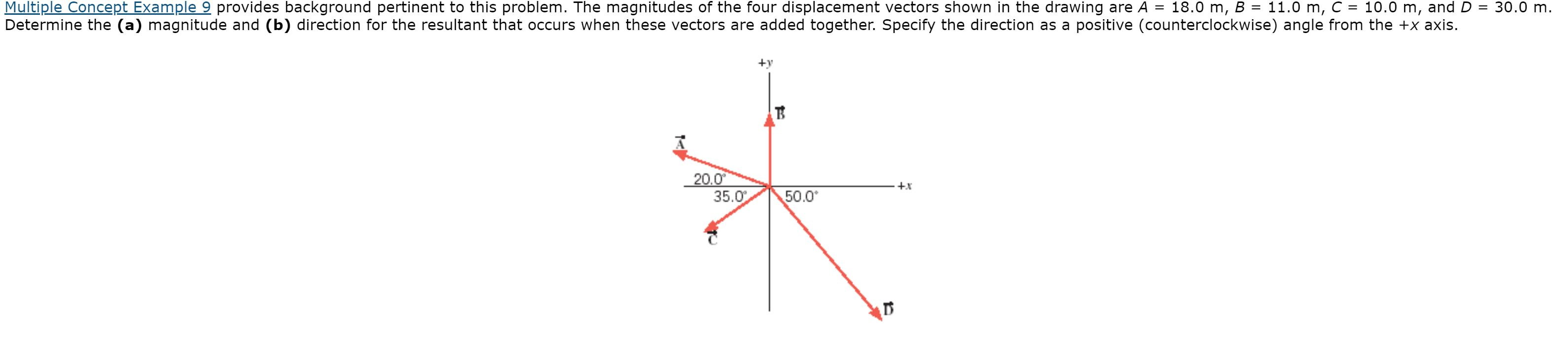# Multiple Concept Example 9 provides background pertinent to this problem. The magnitudes of the four displacement vectors shown in the drawing are A =10.0 m, andD = 30.0 m.18.0 m, B = 11.0 m, C%3DDetermine the (a) magnitude and (b) direction for the resultant that occurs when these vectors are added together. Specify the direction as a positive (counterclockwise) angle from the +x axis.+y20.035.0+x50.0

Question
38 viewshelp_outlineImage TranscriptioncloseMultiple Concept Example 9 provides background pertinent to this problem. The magnitudes of the four displacement vectors shown in the drawing are A = 10.0 m, and D = 30.0 m. 18.0 m, B = 11.0 m, C %3D Determine the (a) magnitude and (b) direction for the resultant that occurs when these vectors are added together. Specify the direction as a positive (counterclockwise) angle from the +x axis. +y 20.0 35.0 +x 50.0 fullscreen
check_circle

Step 1

Write the expression for resultant of the four displacement vectors

Step 2

Substitute the values

Step 3

Find the magnitude of t...

### Want to see the full answer?

See Solution

#### Want to see this answer and more?

Solutions are written by subject experts who are available 24/7. Questions are typically answered within 1 hour.*

See Solution
*Response times may vary by subject and question.
Tagged in

### Vectors and Scalars